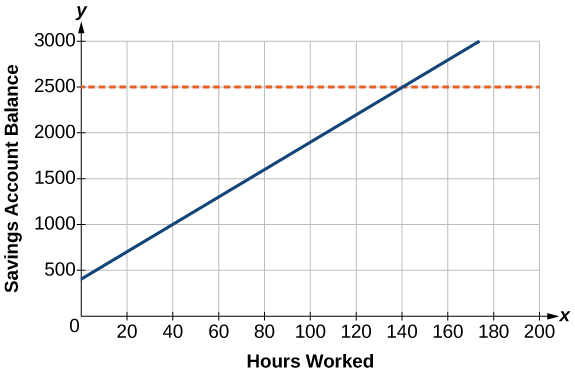## Introduction to Linear Equations in One Variable

### Learning Objectives

By the end of this section, you will be able to:

• Solve equations in one variable algebraically.
• Solve a rational equation.
• Find a linear equation.
• Given the equations of two lines, determine whether their graphs are parallel or perpendicular.
• Write the equation of a line parallel or perpendicular to a given line.

Caroline is a full-time college student planning a spring break vacation. To earn enough money for the trip, she has taken a part-time job at the local bank that pays $15.00/hr, and she opened a savings account with an initial deposit of$400 on January 15. She arranged for direct deposit of her payroll checks. If spring break begins March 20 and the trip will cost approximately \$2,500, how many hours will she have to work to earn enough to pay for her vacation? If she can only work 4 hours per day, how many days per week will she have to work? How many weeks will it take? In this section, we will investigate problems like this and others, which generate graphs like the line in Figure 1.Figure 1